Traveltime approximations for transversely isotropic media with an inhomogeneous backgroundNext: Appendix C: The homogeneous Up: Alkhalifah: TI traveltimes in Previous: Appendix A: Expansion in

# Appendix B: Expansion inandFor an expansion inand, simultaneously, I use the following trial solution:(30)

in terms of the coefficients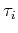, where thecorresponds to, and. Inserting the trial solution, equation B-1, into equation A-1 yields again a long formula, but by setting bothand, I obtain the zeroth-order term given by(31)

which is simply the eikonal formula for elliptical anisotropy. By equating the coefficients of the powers of the independent parameterand, in succession starting with first powers of the two parameters, we end up first with the coefficients of first-power inand zeroth power in, simplified by using equation B-2, and given by(32)

which is a first-order linear partial differential equation in. The coefficients of zero-power inand the first-power inis given by(33)

The coefficients of the square terms in, with some manipulation, results in the following relation(34)

which is again a first-order linear partial differential equation inwith an obviously more complicated source function given by the right hand side. The coefficients of the square terms in, with also some manipulation, results in the following relation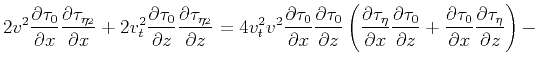(35)

which is again a first-order linear partial differential equation inwith a again complicated source function.

Finally, the coefficients of the first-power terms in bothandresults also in a first-order linear partial differential equation in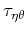given by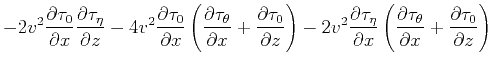(36)

Though the equation seems complicated, many of the variables of the source function (right hand side) can be evaluated during the evaluation of equations B-3 and B-4 in a fashion that will not add much to the cost.

Using Shanks transforms (Bender and Orszag, 1978) we can isolate and remove the most transient behavior of the expansion B-1 in(theexpansion did not improve with such a treatment) by first defining the following parameters: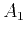(37)

The first sequence of Shanks transforms uses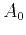,, and, and thus, is given by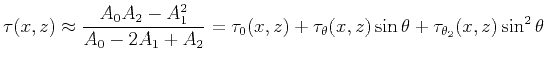(38)Traveltime approximations for transversely isotropic media with an inhomogeneous backgroundNext: Appendix C: The homogeneous Up: Alkhalifah: TI traveltimes in Previous: Appendix A: Expansion in

2013-04-02